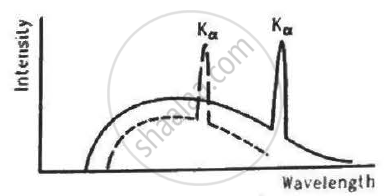Department of Pre-University Education, KarnatakaPUC Karnataka Science Class 12
Share

# The Figure Shows the Intensity-wavelength Relations of X-rays Coming from Two Different Coolidge Tubes. the Solid Curve Represents the Relation for the Tube a in Which the Potential Difference - Physics

ConceptElectromagnetic Spectrum

#### Question

The figure shows the intensity-wavelength relations of X-rays coming from two different Coolidge tubes. The solid curve represents the relation for the tube A in which the potential difference between the target and the filament is VA and the atomic number of the target material is ZA. These quantities are VB and ZB for the other tube. Then,• VA > VB, ZA > ZB

• VA > VB, ZA < ZB

• VA < VB, ZA > ZB

• VA > VB, ZA < ZB

#### Solution

VA > VB, ZA < ZB

It is clear from the figure that the X-ray of tube A has less cutoff wavelength than the X-ray of tube B.

therefore lambda_A < lambda_B

Using Moseley's Law,

Z_A < Z_B

lambda ∝ 1/V ,  where V is the voltage applied in the X-ray tube.

therefore V_A > V_B

Is there an error in this question or solution?

#### Video TutorialsVIEW ALL 

Solution The Figure Shows the Intensity-wavelength Relations of X-rays Coming from Two Different Coolidge Tubes. the Solid Curve Represents the Relation for the Tube a in Which the Potential Difference Concept: Electromagnetic Spectrum.
S